• 文章目录引言一、分类与预测1.常用的分类与预测算法2....数据挖掘实战—餐饮行业的数据挖掘之数据探索(EDA) 数据挖掘实战—餐饮行业的数据挖掘之数据预处理 引言   经过数据探索与数据预处理，我们得
文章目录引言一、分类与预测1.常用的分类与预测算法2. 回归分析3.决策树4.人工神经网络二、聚类分析1.常用的聚类分析算法2.K-Means聚类算法2.1 算法过程2.2 数据类型与相似性的度量3. 目标函数4.消费行为特征分析5.python中主要的聚类分析算法三、关联规则1.常用的关联规则算法2.Apriori算法2.1.Apriori的性质2.2.Apriori算法实现过程2.3.Apriori算法实现过程实例3.Apriori算法—python实现四、时间序列模式五、离群点检测1.离群点的成因及分类2.离群点检测方法3.基于聚类的离群点检测方法3.1 丢弃远离其他簇的小簇3.2 基于原型的聚类

本文参考《python数据分析与挖掘实战》
数据集链接，密码1234
《python数据分析与挖掘实战》pdf链接，密码1234
数据挖掘实战—餐饮行业的数据挖掘之数据探索(EDA)
数据挖掘实战—餐饮行业的数据挖掘之数据预处理

引言
经过数据探索与数据预处理，我们得到了可以直接建模的数据，根据挖掘目标与数据形式我们可以建立分类与预测、聚类分析、关联规则、时序模式、离群点检测等模型，帮助企业提取数据中蕴含的商业价值。
一、分类与预测
对于餐饮行业，常遇到如下问题：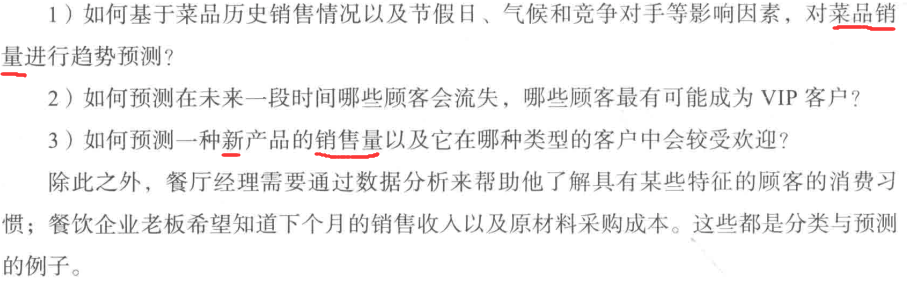1.常用的分类与预测算法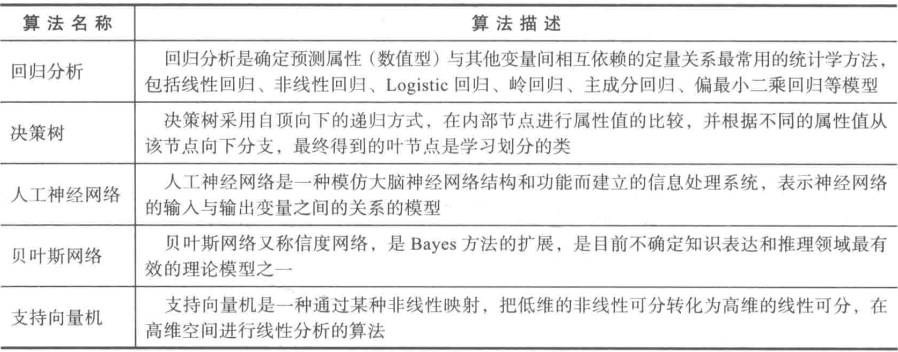2. 回归分析
在数据挖掘环境下。自变量与因变量具有相关关系，自变量是已知的，因变量是要预测的。常见的回归模型如下：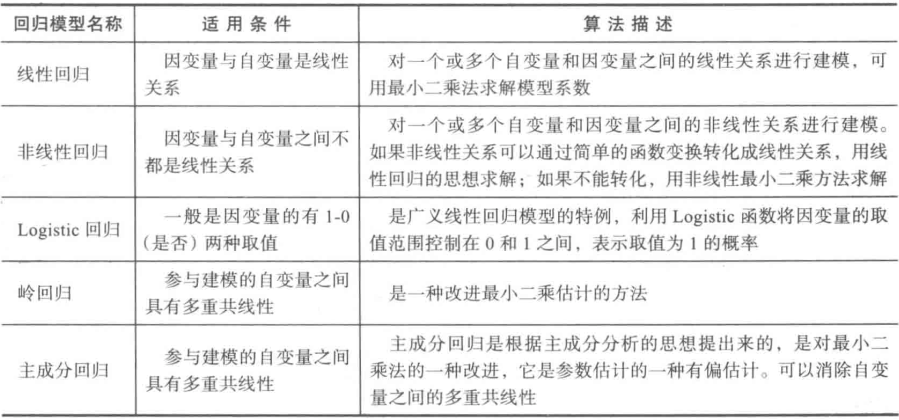当自变量之间出现多重共线性时，用最小二乘法估计回归系数将不准确，消除多重共线性的参数改进方法主要有岭回归和主成分回归
3.决策树
决策树是一种树状结构，它的每一个叶结点对应着一个分类，非叶结点对应着在某个属性上的划分。根据样本在该属性上的不同取值将划分成若干子集。对于非纯叶节点，多数类的标号给出到达这个节点的样本所属的类。构造决策树的核心问题是在每一步如何选择适当的属性对样本进行拆分。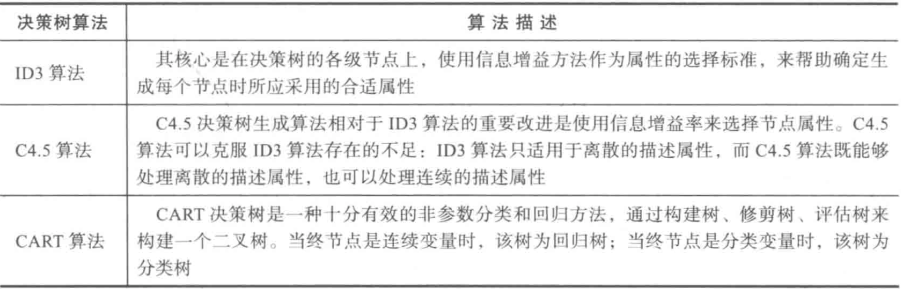#!usr/bin/env python
# -*- coding:utf-8 -*-
"""
@file: ID3决策树.py
@time: 2021/03/31
@desc:
"""
import pandas as pd
from sklearn.tree import DecisionTreeClassifier, export_graphviz
from sklearn.preprocessing import LabelEncoder
import graphviz
import matplotlib as mpl

data[data == '好'] = 1
data[data == '高'] = 1
data[data == '是'] = 1
data[data != 1] = -1
x = data.iloc[:, :3].values.astype(int)
y = data.iloc[:, 3].values.astype(int)
dtc = DecisionTreeClassifier(criterion='entropy')
dtc.fit(x, y)

dot_data = export_graphviz(dtc, out_file='tree.dot',
feature_names=['天气', '是否周末', '是否有促销'],
class_names=['高', '低'], filled=True,
rounded=True, special_characters=True)

with open('tree.dot', encoding='utf-8') as f:
dot = graphviz.Source(dot_grapth.replace("helvetica", "MicrosoftYaHei"))   # 解决中文乱码replace("helvetica", "MicrosoftYaHei")
dot.view()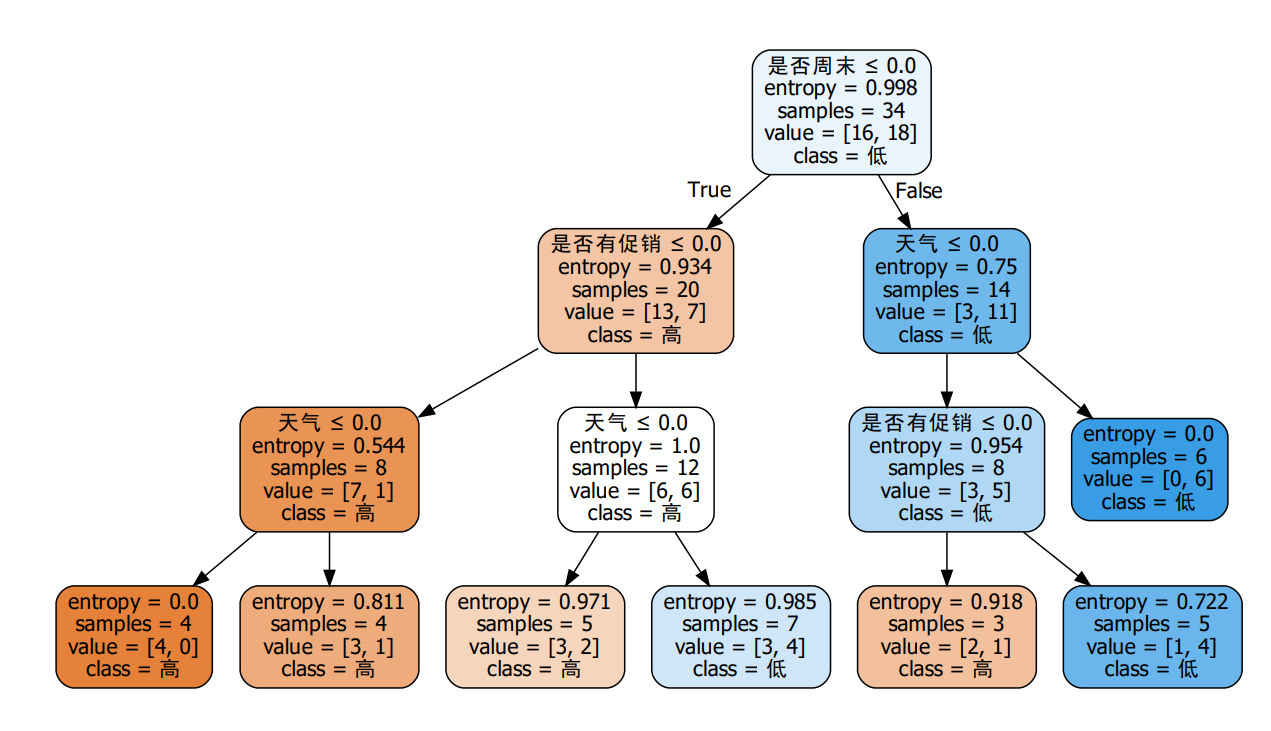4.人工神经网络
使用人工神经网络模型需要确定网络连接的拓扑结构、神经元的特征和学习规则。常用来实现分类与预测的人工神经网络算法有：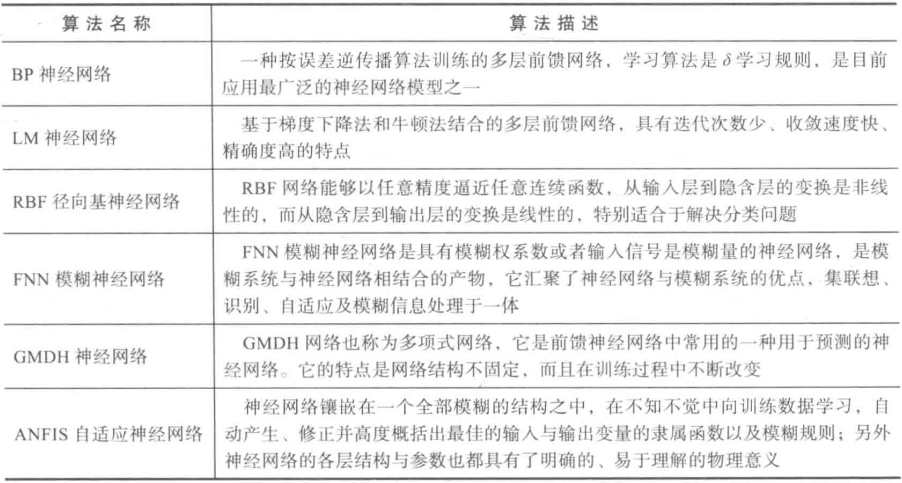import pandas as pd
import tensorflow as tf
from tensorflow import keras
from tensorflow.keras import layers, Model, optimizers, losses, metrics, activations
from tensorflow.keras.layers import Dense, Dropout, Input, Lambda
import matplotlib as mpl

data[data == '好'] = 1
data[data == '高'] = 1
data[data == '是'] = 1
data[data != 1] = 0
x = data.iloc[:, :3].values.astype(int)
y = data.iloc[:, 3].values.astype(int)

def bpModel():
input = Input(shape=(3,))
x = Dense(10, activation=tf.nn.relu)(input)
x = Dense(10, activation=tf.nn.relu)(x)
output = Dense(1, activation='sigmoid')(x)

model = Model(inputs=input, outputs=output)
loss='binary_crossentropy',
metrics=['accuracy'])
return model

def cm_plot(y, yp):
"""
混淆矩阵可视化
:param y:
:param yp:
:return:
"""
from sklearn.metrics import confusion_matrix

cm = confusion_matrix(y, yp)
print(cm)
import matplotlib.pyplot as plt
plt.matshow(cm, cmap=plt.cm.Greens)
plt.colorbar()

for x in range(len(cm)):
for y in range(len(cm)):
plt.annotate(cm[x, y], xy=(x, y), horizontalalignment='center', verticalalignment='center')

plt.ylabel('True label')
plt.xlabel('Predicted label')
return plt

bp = bpModel()
bp.fit(x, y, epochs=1000, batch_size=10)
y_pred = bp.predict(x)
y_pred[y_pred >= 0.5] = 1
y_pred[y_pred < 0.5] = 0

# 可视化
cm_plot(y, y_pred).show()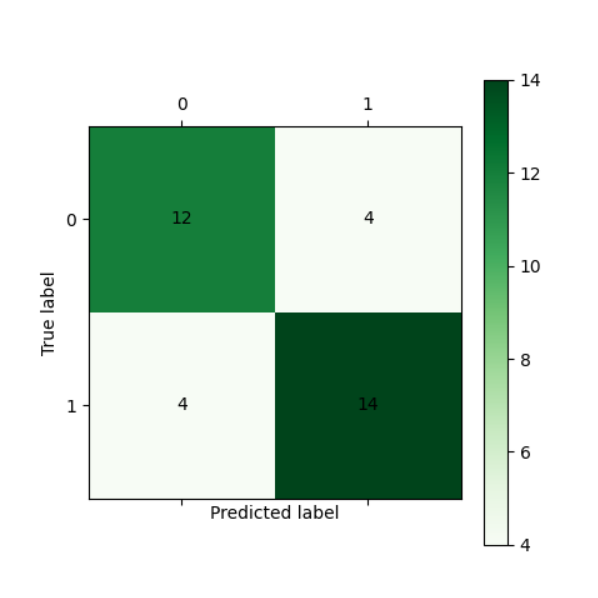二、聚类分析
在当前市场环境下，消费者需求显现出日益差异化和个性化的趋势。针对餐饮行业，常碰到以下问题：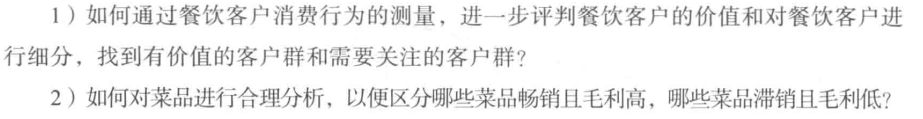这些问题都可以通过聚类分析来实现。
1.常用的聚类分析算法
聚类方法是一种无监督的学习方法。在一组未被标记的样本上，聚类根据数据自身的距离或相似度将它们划分为若干组。划分的原则是组内样本最小化而组间样本距离最大化。
常用聚类方法：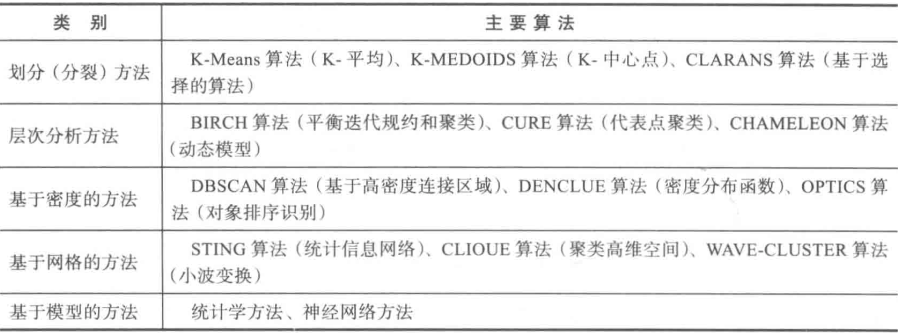常用聚类分析方法：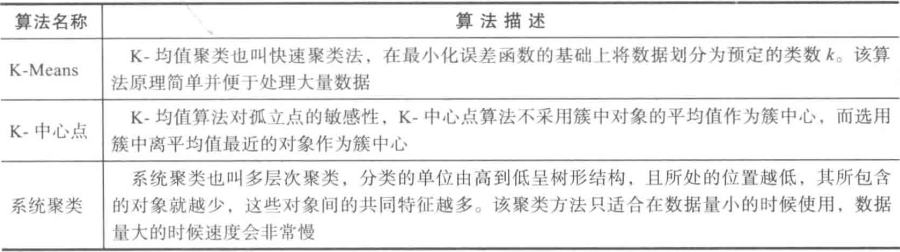2.K-Means聚类算法
K-Means聚类算法是典型的基于距离的非层次聚类算法，在最小化误差函数的基础上将数据划分为预定的类数$K$，采用距离作为相似性的评价指标，即认为两个对象距离越近，其相似度越大。
2.1 算法过程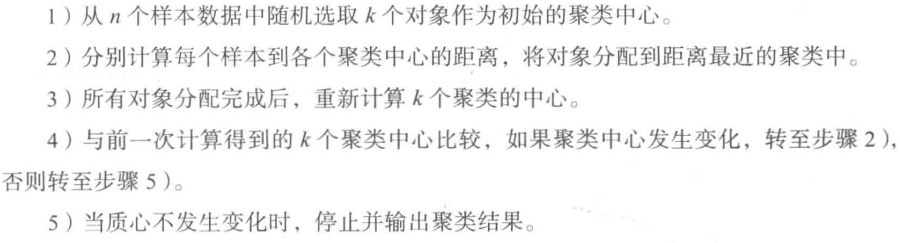聚类的结果依赖于初始聚类中心的随机选择，有可能使得结果严重偏离全局最优分类。实际中，多次运行K-Means算法，选择误差平方和最小的聚类结果。
2.2 数据类型与相似性的度量
对于连续属性，要先对各属性值进行标准化，再进行距离计算。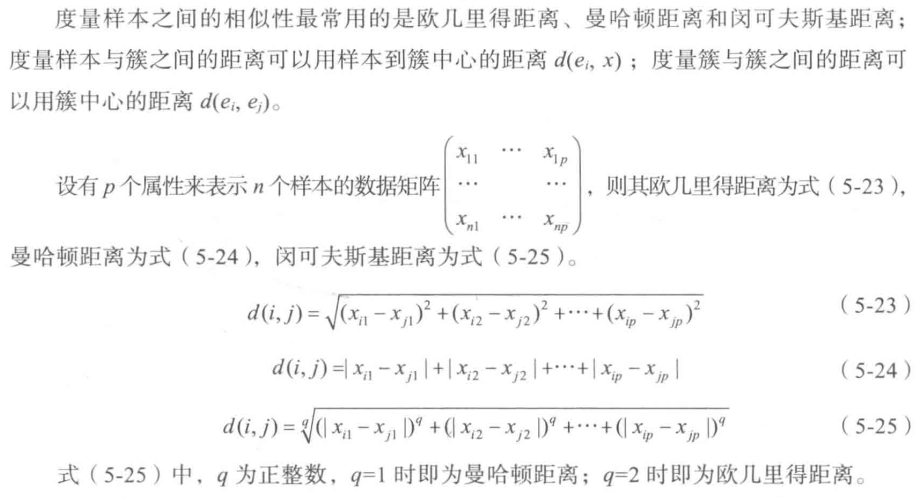对于文档数据，可使用余弦相似性。可先将文档数据转换成文档—词矩阵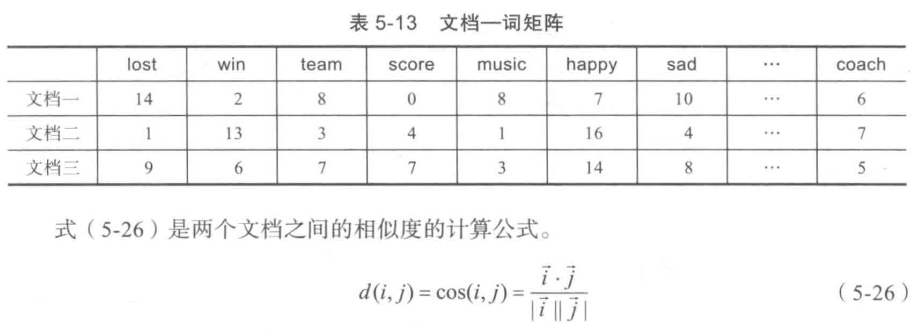3. 目标函数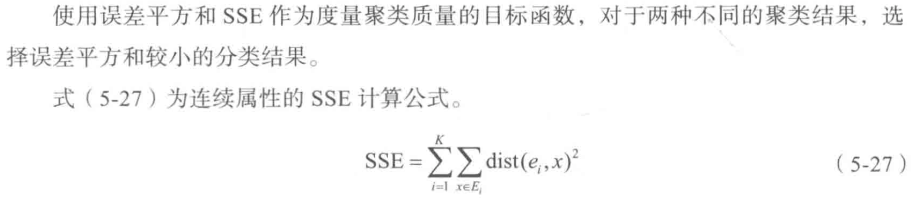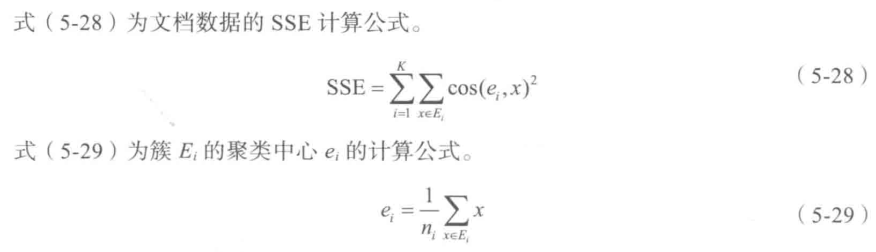4.消费行为特征分析
以部分餐饮客户消费行为特征数据为例，聚类分析得
import pandas as pd
import numpy as np
from sklearn.cluster import KMeans
from sklearn.preprocessing import StandardScaler

# 加载数据
# 连续属性标准化
ss = StandardScaler()
x = ss.fit_transform(data)

k = 3  # 聚类中心数
n_iterations = 500  # 聚类的最大循环次数
kmeans = KMeans(n_clusters=k, max_iter=n_iterations, n_jobs=-1, random_state=1234)
kmeans.fit(data)  # 训练

# 统计各类别的数目
r1 = pd.Series(kmeans.labels_).value_counts()
# 找出聚类中心
r2 = pd.DataFrame(kmeans.cluster_centers_)
# 拼接,得到各聚类中心下类别的数目
r = pd.concat([r2, r1], axis=1)
# 重命名表头
r.columns = list(data.columns) + ['类别数目']

# 将聚类类别保存到数据中
data = pd.concat([data, pd.Series(kmeans.labels_, index=data.index)], axis=1)
data.to_excel('data/data_type.xls')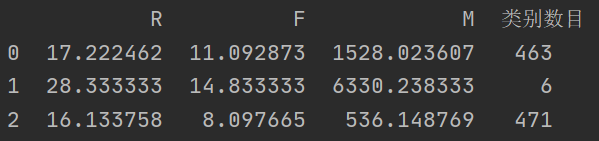绘制聚类后的概率密度图
import pandas as pd
import numpy as np
from sklearn.cluster import KMeans
from sklearn.preprocessing import StandardScaler
import matplotlib.pyplot as plt

# 加载数据
# 连续属性标准化
ss = StandardScaler()
x = ss.fit_transform(data)

k = 3  # 聚类中心数
n_iterations = 500  # 聚类的最大循环次数
kmeans = KMeans(n_clusters=k, max_iter=n_iterations, n_jobs=-1, random_state=1234)
kmeans.fit(data)  # 训练

# 统计各类别的数目
r1 = pd.Series(kmeans.labels_).value_counts()
# 找出聚类中心
r2 = pd.DataFrame(kmeans.cluster_centers_)
# 拼接,得到各聚类中心下类别的数目
r = pd.concat([r2, r1], axis=1)
# 重命名表头
r.columns = list(data.columns) + ['类别数目']
print(r)
# 将聚类类别保存到数据中
rs = pd.concat([data, pd.Series(kmeans.labels_, index=data.index)], axis=1)
rs.columns = list(data.columns) + ['聚类类别']
rs.to_excel('data/data_type.xls')

# 解决中文字体问题
plt.rcParams['font.sans-serif'] = [u'simHei']
plt.rcParams['axes.unicode_minus'] = False

def density_plot(data1):
p = data1.plot(kind='kde', linewidth=2, subplots=True, sharex=False)
[p[i].set_ylabel(u'密度') for i in range(3)]
plt.legend()
return plt

# 概率密度图文件名前缀
pic_output = './data/pd'
for i in range(k):
density_plot(data[rs[u'聚类类别'] == i]).show()
# density_plot(data[rs[u'聚类类别'] == i]).savefig(u'%s%s.png' %(pic_output, i))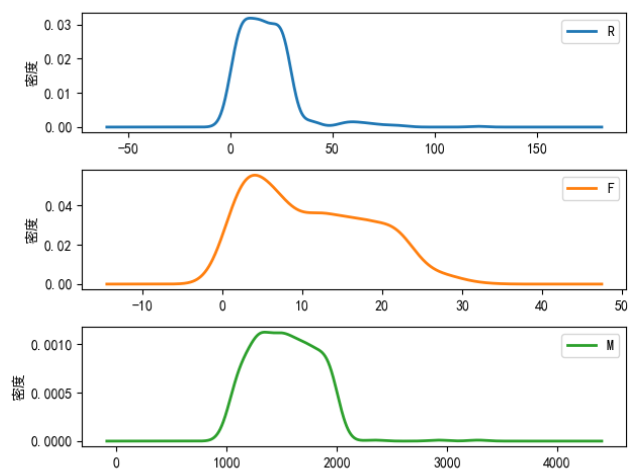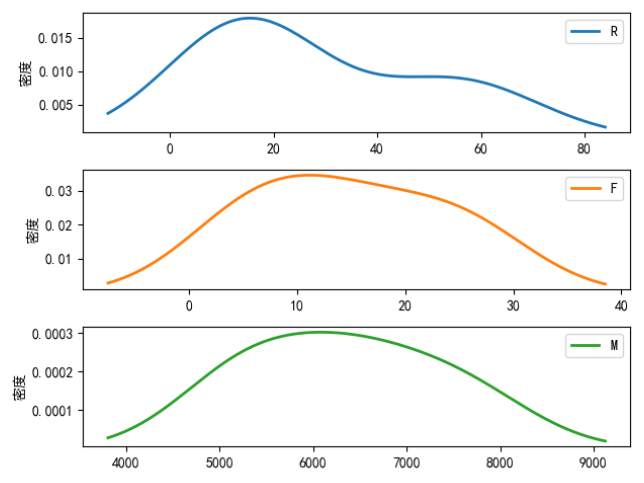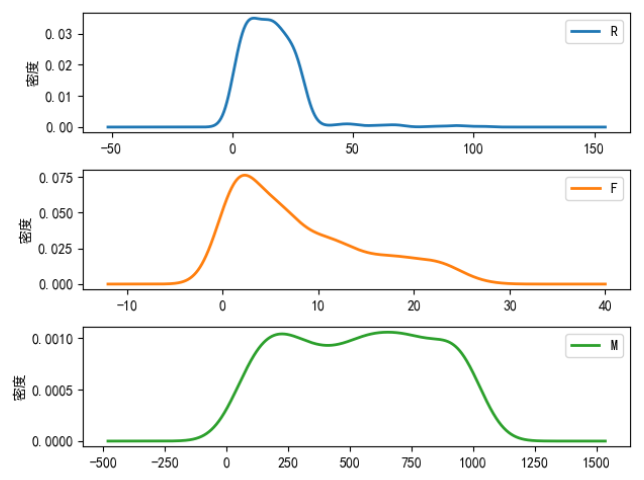5.python中主要的聚类分析算法
scikit-learn库中实现的聚类主要有：K-Means聚类、层次聚类、FCM以及神经网络聚类。主要相关函数如下表：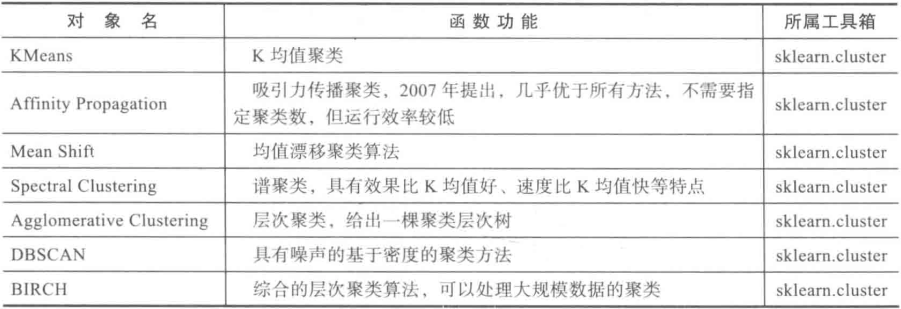三、关联规则
关联规则分析是数据挖掘中最活跃的研究方法之一，目的是在一个数据集中找到各项之间的关联关系，而这种关系并没有在数据中直接体现出来。在餐饮行业中，菜品的推荐就用到了关联规则，比如某些菜品是相互关联的，有些菜品之间是竞争关系，在顾客下单时推荐相关联的产品就可以引导顾客消费。
1.常用的关联规则算法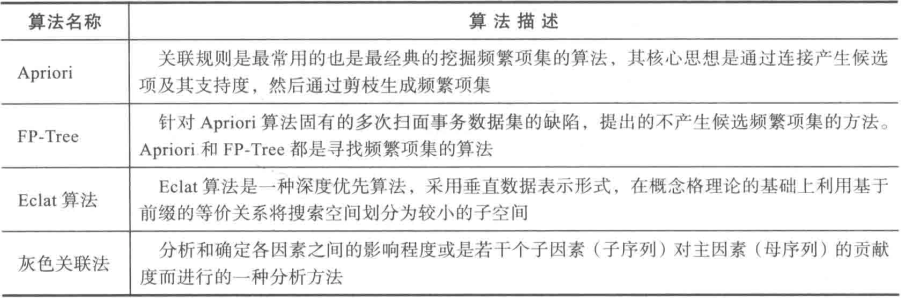2.Apriori算法
Apriori算法的主要思想是找出存在于事务数据集中最大的频繁项集，再利用得到的最大频繁项集与预先设定的最小置信度阈值生成强关联规则。
2.1.Apriori的性质
频繁项集的所有非空子集一定是频繁项集。根据这一性质可以得出：向不是频繁项集$I$的项集中添加事务$A$，新的项集$I∪A$一定不是频繁项集。
2.2.Apriori算法实现过程
第一步：
找出所有频繁项集（支持度必须大于等于给定的最小支持度阈值），在这个过程中连接步与剪枝步相互融合，最终得到最大频繁项集$L_k$

连接步
连接步的目的是找到$K$项集。对于给定的最小支持度阈值，分别对1项候选集$C_1$，剔除小于该阈值的项集得到1项频繁集$L_1$;下一步由$L_1$自身连接产生2项候选集$C_2$，剔除小于该阈值的项集得到2项频繁集$L_2$；再下一步由$L_2和L_1$连接产生3项候选集$C_3$，剔除小于该阈值的项集得到3项频繁集$L_3$，这样循环下去，直至由$L_{k-1}和L_1$连接产生$k$项候选集$C_k$，剔除小于该阈值的项集得到最大频繁集$L_k$
剪枝步
剪枝步紧接着连接步，在产生候选项$C_k$的过程中起到了减小搜索空间的目的。根据Apriori的性质：频繁项集的所有非空子集也必须是频繁项集，所以不满足该性质的项集将不会存在于$C_k$中，该过程就是剪枝

第二步：
由频繁项集产生强关联规则。由第一步可知，未超过预定的最小支持阈值的项集已被剔除，如果剩下的这些项集又满足了预定的最小置信度阈值，那么就挖掘出了强关联规则。
2.3.Apriori算法实现过程实例
以餐饮行业点餐数据为例，首先先将事务数据整理成关联规则模型所需的数据结构。设最小支持度为0.2，将菜品id编号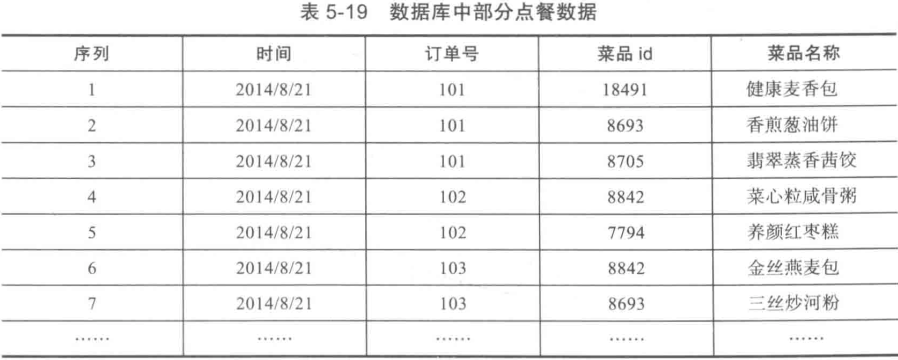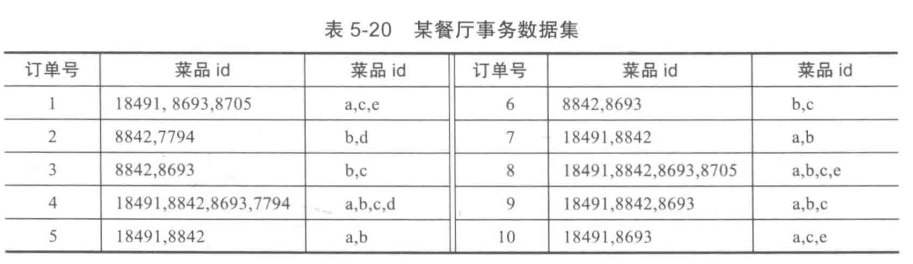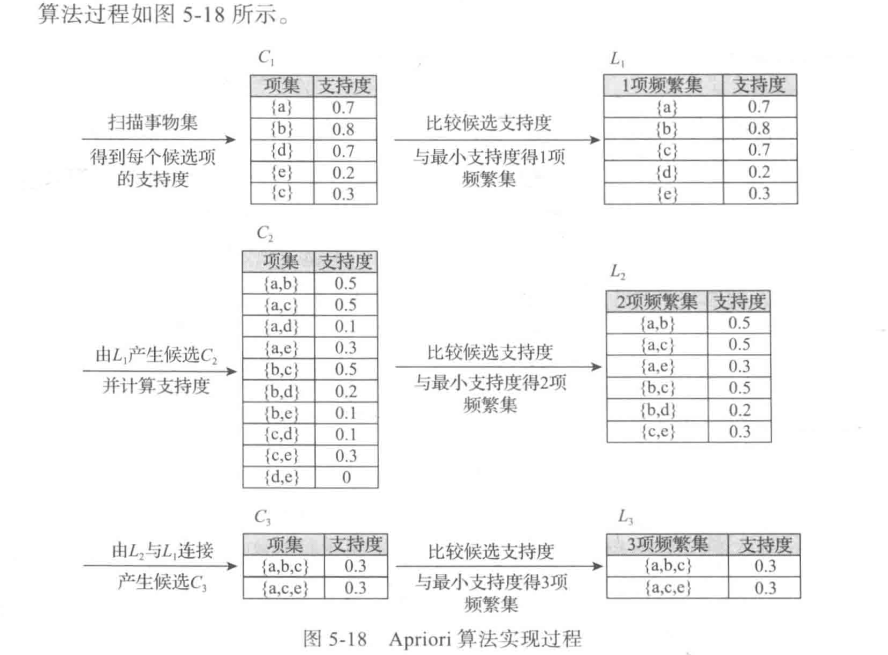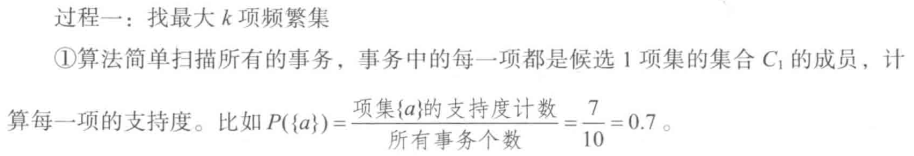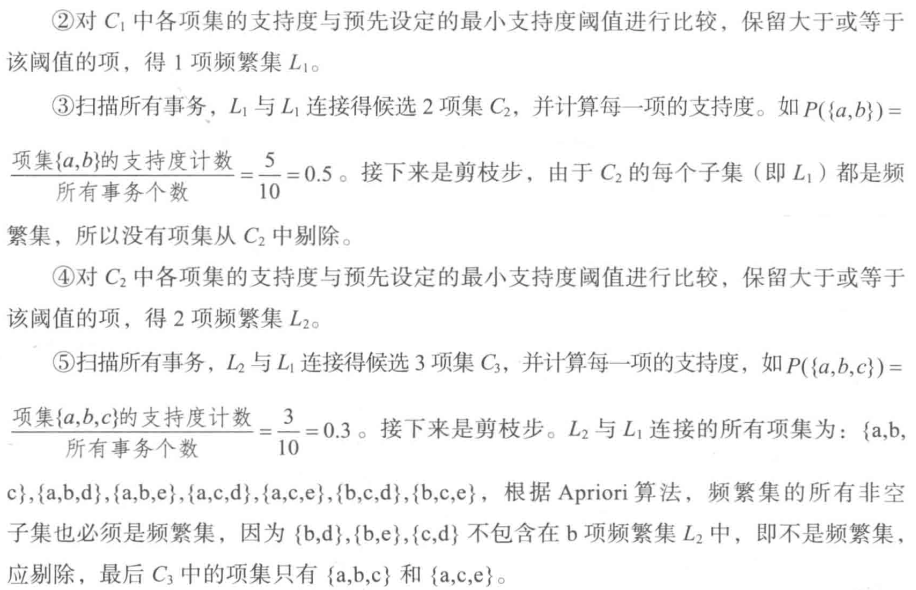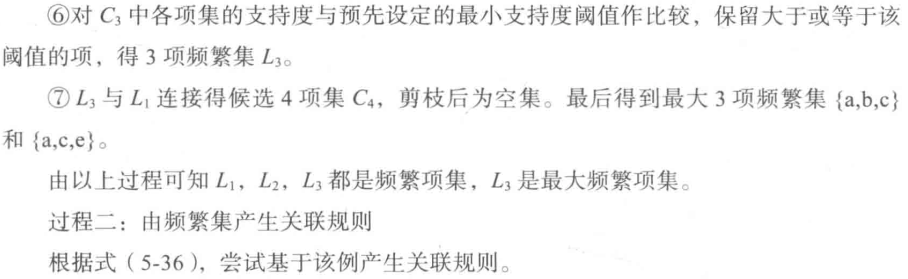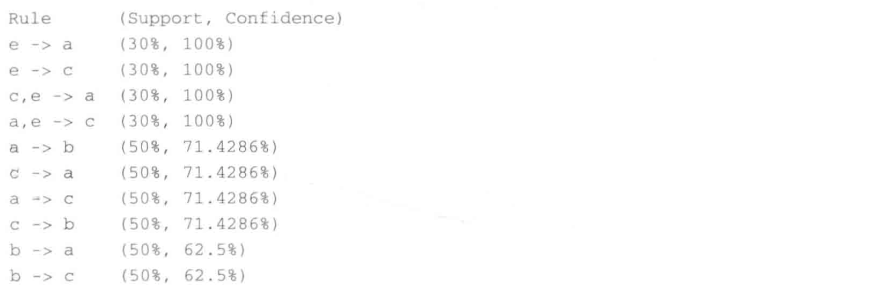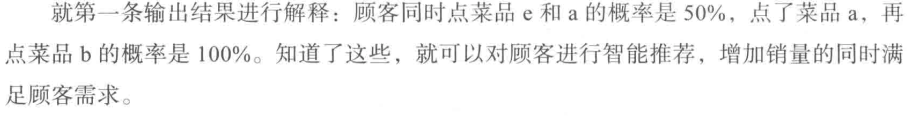3.Apriori算法—python实现
数据集链接
import numpy as np
import pandas as pd

def connect_string(x, ms):
"""
与1项频繁集连接生成新的项集
:param x: 项集
:param ms:
:return: 新的项集
"""
x = list(map(lambda i: sorted(i.split(ms)), x))
l = len(x)
r = []
for i in range(len(x)):
for j in range(i, len(x)):
if x[i][:l - 1] == x[j][:l - 1] and x[i][l - 1] != x[j][l - 1]:
r.append(x[i][:l - 1] + sorted([x[j][l - 1], x[i][l - 1]]))
return r

def find_rule(d, support, confidence, ms=u'-'):
"""
寻找关联规则
:param d: 数据集
:param support: 最小支持度
:param confidence: 最小置信度
:param ms: 项集之间连接符号
:return: 强关联规则以及其支持度与置信度
"""
# 存储输出结果
result = pd.DataFrame(index=['support', 'confidence'])

# 1项集的支持度序列
support_series = 1.0 * d.sum(axis=0) / d.shape
# 基于给定的最小支持度进行筛选,得到1项频繁集
column = list(support_series[support_series > support].index)

# 当1项频繁集个数大于1时
k = 0
while len(column) > 1:
k = k + 1
print(u'\n正在进行第%s次搜索...' % k)
column = connect_string(column, ms)
print(u'数目：%s...' % len(column))
# 乘积为1表示两个项集同时发生，乘积为0表示不同发生
sf = lambda i: d[i].prod(axis=1, numeric_only=True)  # 新一批支持度的计算函数

# 创建连接数据，这一步耗时、耗内存最严重。当数据集较大时，可以考虑并行运算优化。
d_2 = pd.DataFrame(list(map(sf, column)), index=[ms.join(i) for i in column]).T

# 计算连接后的支持度
support_series_2 = 1.0 * d_2[[ms.join(i) for i in column]].sum() / len(d)
column = list(support_series_2[support_series_2 > support].index)  # 新一轮支持度筛选
support_series = support_series.append(support_series_2)

column2 = []
# 遍历可能的推理，如{A,B,C}究竟是A+B-->C还是B+C-->A还是C+A-->B？
for i in column:
i = i.split(ms)
for j in range(len(i)):
column2.append(i[:j] + i[j + 1:] + i[j:j + 1])

# 定义置信度序列
cofidence_series = pd.Series(index=[ms.join(i) for i in column2])
# 计算置信度序列
for i in column2:
cofidence_series[ms.join(i)] = support_series[ms.join(sorted(i))] / support_series[ms.join(i[:len(i) - 1])]

for i in cofidence_series[cofidence_series > confidence].index:  # 置信度筛选
result[i] = 0.0
result[i]['confidence'] = cofidence_series[i]
result[i]['support'] = support_series[ms.join(sorted(i.split(ms)))]

result = result.T.sort_values(['confidence', 'support'], ascending=False)  # 结果整理，输出
print(u'\n结果为：')
print(result)
return result

if __name__ == '__main__':
# 加载数据
print('转换原数据到0-1矩阵')
ct = lambda x: pd.Series(1, index=x[pd.notnull(x)])
b = map(ct, data.values)
data = pd.DataFrame(list(b)).fillna(0)
# 删除中间变脸b
del b

support = 0.2  # 最小支持度
confidence = 0.5  # 最小置信度

find_rule(data, support, confidence)

转换原数据到0-1矩阵

正在进行第1次搜索...
数目：6...

正在进行第2次搜索...
数目：3...

正在进行第3次搜索...
数目：0...

结果为：
support  confidence
e-a        0.3    1.000000
e-c        0.3    1.000000
c-e-a      0.3    1.000000
a-e-c      0.3    1.000000
c-a        0.5    0.714286
a-c        0.5    0.714286
a-b        0.5    0.714286
c-b        0.5    0.714286
b-a        0.5    0.625000
b-c        0.5    0.625000
a-c-e      0.3    0.600000
b-c-a      0.3    0.600000
a-c-b      0.3    0.600000
a-b-c      0.3    0.600000

其中，'e—a’表示e发生能够推出a发生，置信度为100%，支持度30%。搜索出的关联规则并不一定有实际意义，需要根据问题背景筛选适当的有意义的规则，并赋予合理的解释。
四、时间序列模式
见这个博客
五、离群点检测
离群点检测是数据挖掘中重要的一部分，它的任务是发现与大部分其他对象显著不同的对象。大部分数据挖掘方法都将这种差异信息视为噪声而丢弃。同时，一些噪声数据中，罕见的数据可能蕴含着更大的研究价值。
1.离群点的成因及分类
离群点的主要成因是：数据来源于不同的类、自然变异、数据测量和收集误差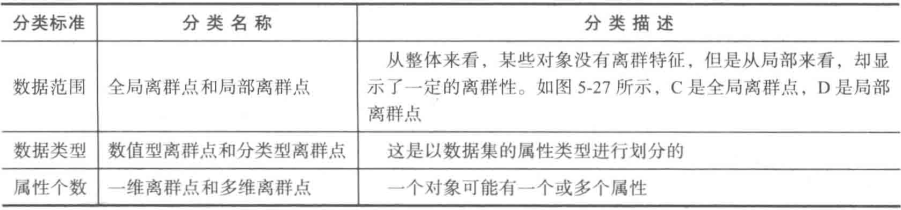2.离群点检测方法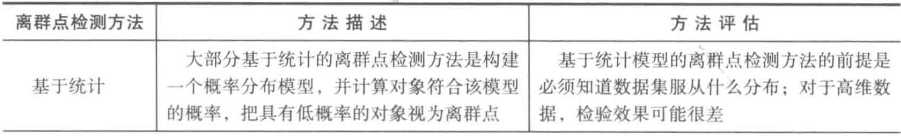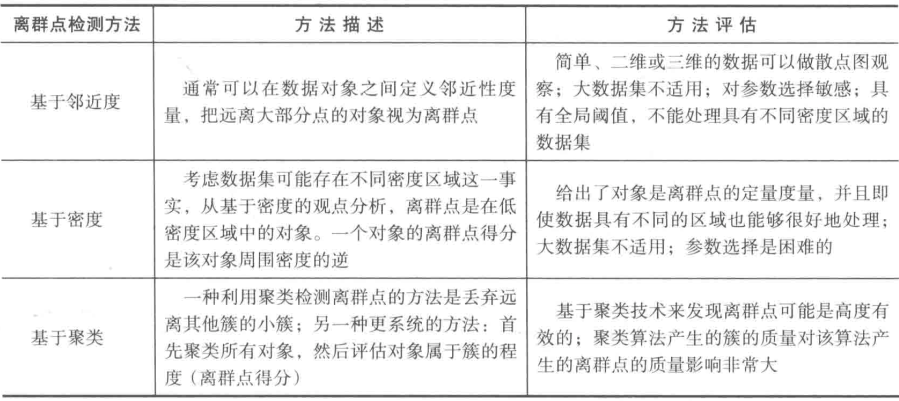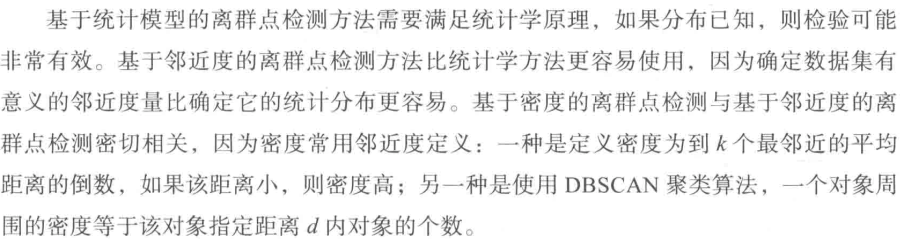3.基于聚类的离群点检测方法
聚类分析用于发现局部强相关的对象组，而异常检测用于发现不与其他对象强相关的对象。因此聚类分析也可以用于离群点检测。下面介绍两种基于聚类的离群点检测方法。
3.1 丢弃远离其他簇的小簇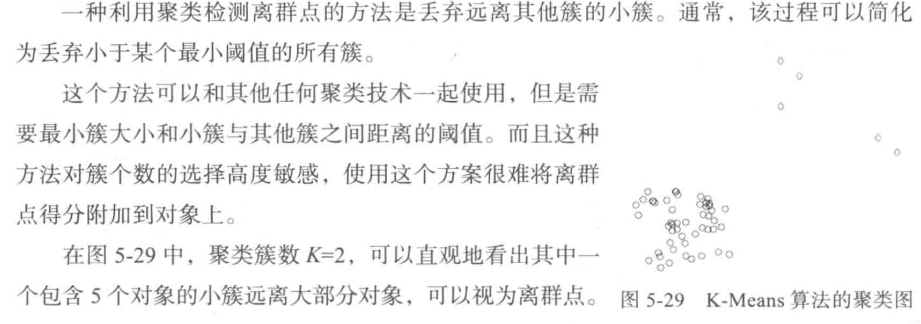3.2 基于原型的聚类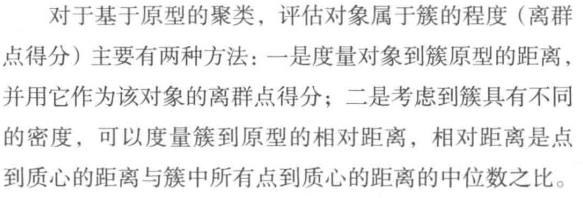下面使用第二种
import numpy as np
import pandas as pd

# 参数初始化
inputfile = '../data/consumption_data.xls'  # 销量及其他属性数据
k = 3  # 聚类的类别
threshold = 2  # 离散点阈值
iteration = 500  # 聚类最大循环次数
data = pd.read_excel(inputfile, index_col = 'Id')  # 读取数据
data_zs = 1.0*(data - data.mean())/data.std()  # 数据标准化

from sklearn.cluster import KMeans
model = KMeans(n_clusters = k, n_jobs = 4, max_iter = iteration)  # 分为k类，并发数4
model.fit(data_zs)  # 开始聚类

# 标准化数据及其类别
r = pd.concat([data_zs, pd.Series(model.labels_, index = data.index)], axis = 1)   # 每个样本对应的类别
r.columns = list(data.columns) + ['聚类类别']  # 重命名表头

norm = []
for i in range(k):  # 逐一处理
norm_tmp = r[['R', 'F', 'M']][r['聚类类别'] == i]-model.cluster_centers_[i]
norm_tmp = norm_tmp.apply(np.linalg.norm, axis = 1)  # 求出绝对距离
norm.append(norm_tmp/norm_tmp.median())  # 求相对距离并添加

norm = pd.concat(norm)  # 合并

import matplotlib.pyplot as plt
plt.rcParams['font.sans-serif'] = ['SimHei']  # 用来正常显示中文标签
plt.rcParams['axes.unicode_minus'] = False  # 用来正常显示负号
norm[norm <= threshold].plot(style = 'go')  # 正常点

discrete_points = norm[norm > threshold]  # 离群点
discrete_points.plot(style = 'ro')

for i in range(len(discrete_points)):  # 离群点做标记
id = discrete_points.index[i]
n = discrete_points.iloc[i]
plt.annotate('(%s, %0.2f)'%(id, n), xy = (id, n), xytext = (id, n))

plt.xlabel('编号')
plt.ylabel('相对距离')
plt.show()

参考：

《python分析与数据挖掘实战》

如果对您有帮助，麻烦点赞关注，这真的对我很重要！！！如果需要互关，请评论留言或私信！展开全文• Python数据分析与数据挖掘实战 有一次培训的讲义，仅仅用作记录，下次可以再好好复习一下。。。Python数据分析与数据挖掘实战 有一次培训的讲义，仅仅用作记录，下次可以再好好复习一下。。。
• icrosoft 数据分析与数据挖掘 实战案例 杨大川 dyangminesage 讲师简介 杨大川-迈思奇科技有限公司CT0 微软MP.2019(最有价值专家) 曾任美国硅谷 Annuncio公司首席工程师 招商迪辰产品研发部总经理 现兼任中科院客座...
• Python数据分析与数据挖掘实战【课程概述】本课程主要针对Python数据分析与数据挖掘实战展开培训，艾威培训Python讲师结合自已多年的Python数据分析与数据挖掘实战经验，结合实战上课；让大家快速掌握到相关技能。...


Python数据分析与数据挖掘实战【课程概述】本课程主要针对Python数据分析与数据挖掘实战展开培训，艾威培训Python讲师结合自已多年的Python数据分析与数据挖掘实战经验，结合实战上课；让大家快速掌握到相关技能。【课程长度】3天【学习对象】数据分析人员，各类企业的数据治理部门【学习目标】掌握使用python进行数据分析和挖掘的技术，能够根据数据和业务场景选择合适的分析算法，能够对数据进行可视化编程。【学员要求】有一定的数据分析工具使用经验(包括excel)。【课程大纲】一、数据分析概况数据分析介绍数据分析作用数据分析流程二、Python概况Python语言特点Python中数据分析模块三、数据准备数据类型数据结构数据导入数据导出四、数据处理数据清洗数据抽取数据合并数据计算数据转换五、数据分析基本统计分组分析结构分析分布分析交叉分析矩阵分析RFM分析六、数据挖掘相关分析简单线性回归多重线性回归逻辑回归决策树分析聚类分析因子分析关联规则时间序列分析七、数据可视化饼图散点图折线图柱形图直方图散点图树图地图热力地图艾威培训感谢大家选择艾威的培训，感谢对艾威的长期的支持与信任。艾威国际培训(Avtech Institute of Technology)，源于美国，始于1998.专业从事企业级在职人员技能提升(项目管理、IT管理、IT技术、云计算大数据、需求管理、信息安全与审计，产品管理等培训)与各类国际认证考试提供商；进入中国16年来，已成为众多500强企业指定的培训供应商。2019年艾威培训将会引入更多国际最先进的课程。欢迎关注艾威公众号avtech88源于美国，始于1998项目管理 |需求管理 |IT管理/技术 | 信息安全 |企业架构 |国际认证 | 企业内训展开全文• ## Python数据挖掘实战

千人学习 2017-12-15 12:04:44
购买课程后，可扫码进入学习群，获取唐宇迪老师答疑 Python数据挖掘技术系列视频培训教程基于真实数据集进行案例实战，使用Python数据科学库从数据预处理开始一步步进行数据建模。对于每个案例首先进行流程解读与...机器学习入门 机器学习面试
• 数据挖掘实战 任务一1.任务要求2.操作步骤2.1数据类型的分布2.2 无关特征删除2.3 数据类型转换2.4 缺失值处理2.5 数据集切分3.实现代码 1.任务要求 对数据进行探索和分析 数据类型的分析 无关特征删除 数据类型转换...
数据挖掘实战 任务一1.任务要求2.操作步骤2.1数据类型的分布2.2 无关特征删除2.3 数据类型转换2.4 缺失值处理2.5 数据集切分3.实现代码
1.任务要求
对数据进行探索和分析

数据类型的分析
无关特征删除
数据类型转换
缺失值处理
其他

2.操作步骤
2.1数据类型的分布
共有4754条记录

float
int
object
total

70
13
7
90

需要对object对象进行处理
2.2 无关特征删除
删除部分内容
卡号：‘bank_card_no’
资源：‘source’
客户姓名：‘id_name’
最新查询时间：‘latest_query_time’
最新贷款时间：‘loans_latest_time’
2.3 数据类型转换
产看该类型下特征

一线城市
二线城市
三线城市
境外
其他城市
nan
使用one-hot编码，分别用1，2，3，4，5进行代替

2.4 缺失值处理
删除缺失值超过 30%的特征
对于剩余的缺失值，这里采用 平均值填充法 进行填充
2.5 数据集切分
借助于sklearn进行切分
3.实现代码
import pandas as pd
import numpy as np
from sklearn.model_selection import train_test_split

data.info()
for i in range(0, data.shape):
data = data.dropna(axis=1, thresh = 1000)
data = data.dropna(axis=0, thresh = 75)
data = data.fillna(data.median())
train_data, test_data = train_test_split(newdata, test_size=0.3, random_state=2018)



展开全文• 通过python进行数据挖掘分析，对数据进行分析预测，干货满满分析预测
• 《Python数据分析与数据挖掘实战》学习笔记（上）数据挖掘基础数据取样数据探索一、缺失、异常值分析二、统计量分析数据预处理挖掘建模模型评价Python数据分析工具插入链接与图片如何插入一段漂亮的代码片生成一个...
《Python数据分析与数据挖掘实战》学习笔记（数据挖掘基础）前言1、获取数据（获取【爬虫采集或从一些统计网站下载】--->取样）2、数据探索2.1、缺失、异常值分析2.2、统计量分析3、数据预处理（清洗【去掉脏数据】、集成【集中】、变换【规范化】、规约【精简】）3.1、数据清洗3.2、数据集成3.3、数据变换3.4、数据规约4、挖掘建模（分类、聚类、关联、预测）4.1、分类与预测逻辑回归逻辑回归建模步骤：案例：对银行在降低货款拖欠率的数据进行逻辑回归建模4.2、聚类分析4.3、关联规则4.4、时序模式4.5、离群点检测5、模型评价与分布
前言
数据分析是对已知的数据进行分析，然后提取出一些有价值的信息，数据量不会太大；数据挖掘，是指对大量数据进行分析与挖掘，得到一些未知的，潜在的信息；数据挖掘是数据分析的提升。
数据挖掘是：从历史保存的大量数据（包括文本）中挖掘出隐含的、未知的、对决策有潜在价值的关系、模式和趋势，并用这些知识和规则建立用于决策支持的模型，提供预测性决策支持的方法、工具和过程。它利用各种分析工具在大量数据中寻找其规律和发现模型与数据之间关系的过程，是统计学、数据库技术和人工智能技术的综合。
数据挖掘的基本任务：利用分类与预测、聚类分析、关联规则、时序模式、偏差检测、智能推荐等方法，帮助企业提取数据中的商业价值。
数据挖掘建模过程包括：定义挖掘目标、数据取样、数据探索、数据预处理、挖掘建模、模型评价六个步骤。
1、获取数据（获取【爬虫采集或从一些统计网站下载】—>取样）
数据取样是在明确了挖掘目标后，从数据仓库中抽取一个相关的样本子集。通过对数据样本的精选，不仅能减少数据处理量，节省系统资源，还能是规律更容易凸显。
抽取数据的标准，一是相关性，二是可靠性，三是有效性。数据取样的质量一定要把关，若原始数据有误，则很难挖掘探索出数据的规律性。
抽样方法：
1、随机抽样：数据集中的每组观测值都有相同的被抽样的概率；
2、等距抽样：如100组观测值按5%的比例等距抽样，则取第20,40,60,80,100组这5组观测值；
3、分层抽样：首先将样本总体分成若干层，每层中的观测值都有相同的被选用的概率，但不同层可设定不同概率；
4、从起始顺序抽样：从输入数据集的起始处开始抽样；
5、分类抽样：依据某种属性的取值来选择数据子集。
2、数据探索
数据探索和预处理的目的是为了保证样本数据的质量。
数据探索主要包括：数据质量分析（异常值、缺失值、一致性） 和数据特征分析（分布、对比、统计量、相关性、周期性和贡献度）
2.1、缺失、异常值分析
缺失值的处理分为删除存在缺失记录、对可能值进行插补、不处理三种情况。
异常值也称为离群点。指样本中个别值的数值明显偏离其余的观测值。三种常见的离群点分析方法：
（1）简单统计量分析 ：使用pandas库中的describe()函数，可以查看数据基本参数（平均值、标准差、最大最小值、25%50%75%三个分位数）
（2）3${ \delta}$原则
（3）箱型图分析
餐饮销售额数据异常值检测代码：
import pandas as pd
#定义路径
catering_sale="E:\\《Python数据分析与挖掘实战》源数据和代码\\Python数据分析与挖掘实战\\chapter3\\demo\\data\\catering_sale.xls"
'''
读取Excel文件
catering_sale=r"\catering_sale.xls"
'''
#读取数据，指定“日期”为索引列
'''
查看数据基本情况：均值，标准差，最小、最大值，分位值
print(data.describe())
'''
import matplotlib.pyplot as plt #导入图像库
plt.rcParams['font.sans-serif']=['SimHei'] #用来正常显示中文标签
plt.rcParams['axes.unicode_minus']=False #用来正常显示负号

# 画出箱型图，并把异常数据标出来
plt.figure()
p=data.boxplot(return_type='dict')#画箱型图
x=p['fliers'].get_xdata() #fliter为异常值的标签
y=p['fliers'].get_ydata()
y.sort() #从小到大排序，直接改变原对象

# 用annotate添加注释
# 其中有些相近的点，注释会出现重叠，难以看清，需要技巧来控制
# 以下参数都是经过调试的，需要具体问题具体调试
for i in range(len(x)):
if i>0:
plt.annotate(y[i],xy=(x[i],y[i]),xytext=(x[i]+0.05-0.8/(y[i]-y[i-1]),y[i]))
else:
plt.annotate (y[i],xy=(x[i],y[i]),xytext=(x[i]+0.08,y[i]))

plt.show()
p要指定类型，否则报错不能读取p。故将原句p=data.boxplot( ) 改为 p=data.boxplot(return_type=‘dict’)
2.2、统计量分析
从集中趋势（度量参数：均值、中位数、众数）、离中趋势（极差、标准差、变异系数、四分位数间距）两个方面进行分析
均值对极端值很敏感。可以使用截断均值或中位数来度量数据的集中趋势。
#销售数的统计量情况
import pandas as pd
catering_sale="E:\\《Python数据分析与挖掘实战》源数据和代码\\Python数据分析与挖掘实战\\chapter3\\demo\\data\\catering_sale.xls"
data=data[(data[u'销量']>400)&(data[u'销量']<5000)]
statistics=data.describe()

statistics .loc['range']=statistics .loc['max']-statistics.loc['min']#极差
statistics .loc['var']=statistics .loc['std']/statistics .loc ['mean']#变异系数
statistics .loc['dis']=statistics .loc['75%']-statistics .loc['25%']#四分位数间距

print(statistics)
3、数据预处理（清洗【去掉脏数据】、集成【集中】、变换【规范化】、规约【精简】）
数据预处理包括：数据清洗（缺失值处理、异常值处理）、数据集成（实体识别、冗余属性识别）、数据变换（简单函数变换、规范化、连续属性离散化、属性构造、小波变换）和数据规约（属性规约和数值规约）。
3.1、数据清洗
A.缺失值
在数据探索部分提到，缺失值的处理分为删除存在缺失记录、对可能值进行插补、不处理三种情况。常见的插补方法有：均值/中位数/众数插补，使用固定值，最近邻插补，回归方法，插值法。
插值法是利用已知点建立合适的插值函数，未知值由对应点求出的函数值近似替代。重点学习了拉格朗日插值法和牛顿插值法。
用拉格朗日进行插补：
#自定义列向量插值函数
def ployinterp_column(s,n,k=5):#s为列向量，n为被插值的位置，k为取前后的数据个数，默认为5
y=s[list(range (n-k,n))+list(range(n+1,n+1+k))]
y=y[y.notnull()]#剔除空值
return lagrange(y.index,list(y))(n)#插值并返回插值结果

#逐个元素判断是否需要插值
for i in data.columns:
for j in range(len(data)):
if (data[i].isnull())[j]:#如果为空即插值
data[i][j]=ployinterp_column(data[i],j)

data.to_excel(outputfile)#输出结果，写入文件入文件
B.异常值
异常值处理方式包括：删除、视为缺失值、平均值修正、不处理
3.2、数据集成
A.实体识别
目的是统一不同数据源的矛盾（同名异义，异名同义，单位不统一）。
3.3、数据变换
A.简单函数变换
简单函数变换常用来将不具有正态分布的数据变换成具有正态分布的数据，包括平方、开方、取对数、差分运算等
B.规范化
为了消除指标之间的量纲和取值范围差异的影响，需要进行标准化处理，将数据按比例进行缩放，使之落到特定区域，  便于综合分析。
离差标准化（最小最大规范化）：将数据映射到[0,1]之内，是对原始数据的线性变换。
$x^* = \frac{x-min}{max-min}$
标准差标准化（零-均值规范化）：经处理的数据均值为0，标准差为1。${ \delta}$为原始数据的标准差
$x^* = \frac{x-x{\\mean} }{ \delta}$
小数定标规范化
用三种方法进行数据规范：
#_*_coding:utf-8 _*_
import pandas  as pd
import numpy as np

datafile='E:\\《Python数据分析与挖掘实战》源数据和代码\\Python数据分析与挖掘实战\\chapter4\\demo\\data\\normalization_data.xls'

x1=(data-data.min())/(data.max()-data.min())
x2=(data-data.mean())/data.std()
x3=data/10**np.ceil(np.log10(data.abs().max()))
print(x1,x2,x3)

C.连续属性离散化
等宽离散化、等频离散化、基于聚类分析的方法
用三种方法进行数据离散化：
import pandas as pd

datafile='E:\\《Python数据分析与挖掘实战》源数据和代码\\Python数据分析与挖掘实战\\chapter4\\demo\\data\\discretization_data.xls'
data=data[u'肝气郁结证型系数'].copy()
k=4

#等宽离散化
d1=pd.cut(data,k,labels=range(k))

#等频率离散化
w=[1.0*i/k for i in range(k+1)]
w=data.describe(percentiles=w)[4:4+k+1]
w=w*(1-1e-10)
d2=pd.cut(data,w,labels=range(k))

#聚类离散化
from sklearn.cluster import KMeans
kmodel=KMeans(n_clusters=k,n_jobs=1)
kmodel.fit(data.reshape((len(data),1)))
c=pd.DataFrame(kmodel.cluster_centers_ ).sort_values(0)
w=pd.rolling_mean(c,2).iloc[1:]
w=+list(w)+[data.max()]
d3=pd.cut(data,w,labels=range(k))

def cluster_plot(d,k):
import matplotlib.pyplot as plt
plt.rcParams ['font.sans-serif']=['SimHei']#显示中文标签
plt.rcParams['axes.unicode_minus'] = False#显示负号

plt.figure (figsize= (8,3))
for j in range(0,k):

plt.plot(data[d==j],[j for i in d[d==j]],'o')

plt.ylim(-0.5,k-0.5)
return plt

cluster_plot(d1, k).show()

cluster_plot(d2, k).show()

cluster_plot(d3, k).show()

D.属性构造
利用已有的属性集构造新的属性。
线损率属性构造：
import pandas as pd
inputfile='E:/《Python数据分析与挖掘实战》源数据和代码/Python数据分析与挖掘实战/chapter4/demo/data/electricity_data.xls'
outputfile='E:/代码/Data_A_M/.idea/tmp/electricity_data.xls'

data[u'线损率']=(data[u'供入电量']-data[u'供出电量'])/data[u'供入电量']

data.to_excel(outputfile,index=False)

E.小波变换
小波变换是一种信号分析手段，提供了一种非平稳信号的时频分析手段。
小波变换特征提取代码：
import pandas as pd
import  pywt
inputfile='E:/《Python数据分析与挖掘实战》源数据和代码/Python数据分析与挖掘实战/chapter4/demo/data/leleccum.mat'

signal=mat['leleccum']

coeffs=pywt.wavedec(signal,'bior3.7',level=5)

3.4、数据规约
A.属性规约

属性规约方法
合并属性
逐步向前选择
逐步向后删除
决策树归纳
主成分分析

方法描述
将旧属性合为新属性
从空属性集合开始，每次从原来属性集合选择一个最优属性添加到当前子集，直到无法选出最优或达到阈值为止
每次从原来属性集合选择一个最差属性添加到当前子集…
利用决策树的归纳方法对初始数据进行分类归纳学习，获得一个初始决策树，未出现在该决策树上的属性维为无关属性可删除，便获得较优子集
将相关性很高的变量转化成彼此独立不相关的变量

方法类别
创建新的属性维度
直接删除不相关属性
直接删除不相关属性
直接删除不相关属性
连续属性的数据降维

用主成分分析进行数据降维：
import pandas as pd

inputfile='E:/《Python数据分析与挖掘实战》源数据和代码/Python数据分析与挖掘实战/chapter4/demo/data/principal_component.xls'
outputfile='E:/代码/Data_A_M/.idea/tmp/dimention_reducted.xls'

from sklearn.decomposition import PCA

pca=PCA()
pca.fit(data)
pca.components_#返回模型的各个特征向量
pca.explained_variance_ratio_ #返回各个成分各自的方差百分比(贡献率)

pca=PCA(3)#取前三个主成分，即n_components=3
pca.fit(data)
low_d=pca.transform(data)#用它来降低维度
pd.DataFrame(low_d).to_excel (outputfile)
pca.inverse_transform(low_d)
print(low_d)  
B.数值规约
数值规约方法分为有参数方法和无参数方法两类。

有参数方法
无参数方法

使用一个模型来评估数据，只需存放参数，不需存放实际数据
只需存放实际数据

回归（线性回归和多元回归）
直方图、聚类、抽样

4、挖掘建模（分类、聚类、关联、预测）
建模主要考虑两个问题：第一、本次建模属于哪类问题（分类、聚类、关联规则、时序模式、智能推荐）？第二、选用哪种算法构建模型？
4.1、分类与预测
逻辑回归
逻辑回归建模步骤：
1）根据分析目标设置指标变量（自变量和因变量），然后收集数据，根据收集的数据，对特征再次进行筛选。
2）y取1的概率是$p=P(y=1|X)$ ,取0的概率是$1- p$
用$ln(p/(1-p))$和自变量累出线性回归方程，故居出模型中的回归系数。
3）进行模型检验。模型有效性的检验指标包括：最基本的有正确率，其次有混淆矩阵、ROC曲线、KS值等
4）模型的应用：输入自变量的取值，就可以得到预测变量的值，或者根据预测变量的值去控制自变量的取值
案例：对银行在降低货款拖欠率的数据进行逻辑回归建模
#逻辑回归
import pandas as pd
#初始化参数
datafile='E:\\《Python数据分析与挖掘实战》源数据和代码\\Python数据分析与挖掘实战\\chapter5\\demo\\data\\bankloan.xls'
x=data.iloc[:,:8].values
y=data.iloc[:,8].values

from sklearn.linear_model  import LogisticRegression as LR
from sklearn.linear_model  import RandomizedLogisticRegression  as RLR
rlr=RLR()#建立随机逻辑回归模型，筛选变量
rlr.fit(x,y)#训练模型
rlr.get_support()#获得特征筛选结果
print(u'通过随机逻辑回归模型筛选特征结束。')
print(u'有效特征为：%s'%','.join(data.columns[rlr.get_support(indices=True) ]))
x=data[data.columns[rlr.get_support(indices=True) ]].values

lr=LR()
lr.fit(x,y)
print(u'逻辑回归模型训练结束。')
print(u'模型的平均正确率为：%s'% lr.score(x,y))
4.2、聚类分析
4.3、关联规则
4.4、时序模式
4.5、离群点检测
5、模型评价与分布


展开全文• 数据挖掘实战 （2）：数据预处理 第一部分: 第4章数据预处理 4.1数据清洗 4.1.1缺失值处理 4.1.2异常值处理 4.2数据集成 4.2.1实体识别 4.2.2冗余属性识别 4.3数据变换 4.3.1简单函数变换 4.3.2规范化 4.3.3连续属性...python
• 一种 Python数据分析与应用 2-9章 补充并更改标题中的细节代码 补充课后习题的代码 ...Python数据分析与数据挖掘实战 实战篇6-15章 补充并更改财经杂志中的细节代码， 宣布文中涉及到的内容的完整代码
• 文章目录引言一、数据清洗1.缺失值处理1.1 拉格朗日插值法1.2牛顿差值法2.异常值的处理二、数据集成1.实体识别2.冗余属性识别3.数据变换3.1 简单函数变换3.2 规范化3.2.1 最小-最大...  数据挖掘过程中，海量的数据数据预处理
• 数据挖掘实战——金融风控赛题理解指标回顾功能快捷键合理的创建标题，有助于目录的生成如何改变文本的样式插入链接与图片如何插入一段漂亮的代码片生成一个适合你的列表创建一个表格设定内容居中、居左、居右...大数据
• SAS数据挖掘实战精简 中文版 第一章 数据挖掘概述 第二章 SAS数据挖掘基础 第三章 SAS数据管理 第四章 SAS关联规则挖掘 第五章 SAS聚类分析 第六章 SAS预测模型 第七章 SAS可视化技术 附录 1.常用快捷键 2.常见...
• 基础篇介绍了数据挖掘的基本原理，实战篇介绍了一个个真实案例，通过对案例深入浅出的剖析，使读者在不知不觉中通过案例实践获得数据挖掘项目经验，同时快速领悟看似难懂的数据挖掘理论。读者在阅读过程中，应充分...
• 数据挖掘实战第2章用scikit-learn估计器分类 含源码，含数据，含课本所有解析，十分完整。
• 参与Datawhale数据挖掘实战打卡记录, 旨在记录自己不太懂和和不会的内容. Datawhale的代码和学习资料见https://github.com/datawhalechina/team-learning-data-mining/tree/master/PurchaseAndRedemptionForecast 一...
• 这是一个菜鸟的数据挖掘实战记录，目的是在尽量短的时间内入门数据挖掘，少走弯路。内容上，由于自己的确是菜得不行，所以除了必要的编码外，会重点叙述学习方法和思路，并补充其他学习资料。内容预计分为六篇正文，...机器学习 python
• SAS数据挖掘实战篇【二】 从SAS数据挖掘实战篇【一】介绍完目前的数据挖掘基本概念之外，对整个数据挖掘的概念和应用有初步的认识和宏观的把握之后，我们来了解一下SAS数据挖掘实战篇【二】SAS工具的应用。首先来...
• @[TOC]数据挖掘实战 资金流入流出预测赛题 你好！ 这是你第一次使用 Markdown编辑器 所展示的欢迎页。如果你想学习如何使用Markdown编辑器, 可以仔细阅读这篇文章，了解一下Markdown的基本语法知识。 背景介绍 蚂蚁...
• 1. 大数据挖掘实战平台建设是培养云计算与大数据时代新型数据分析人才的需要。 2. 大数据挖掘实战平台建设能有力促进及推动学校及各学院科研水平，为大数据的分析及挖掘提供基础平台。 3. 该工程与实战中心的建立能...
• 案例来自《python数据分析与挖掘实战》 数据集可以到天池下载 背景 问题 企业偷漏税泛滥，影响国家经济基础 ...类似：数据挖掘实战：电力窃漏电用户自动识别 准备工作 数据集下载：python_d...
• Python数据挖掘实战 计算机博士，专注于机器学习与计算机视觉领域，深度学...
• 基于SAS的数据挖掘实例教程，有代码有理论，适合SAS和数据挖掘新手。
• 本文参考《python数据分析与挖掘实战》 引言   数据挖掘的基本任务包括利用分类与预测、聚类分析、关联规则、时序模式、偏差检测、智能推荐等方法，帮助企业提取数据中蕴含的商业价值，提高企业的竞争力。   ...
• ## sas 数据挖掘实战

千次阅读 2019-05-11 07:31:10
最近学习了SAS数据挖掘，在这里算是做个总结。 实验配置：WIN10 SAS9.2 企业数据挖掘 数据：百度云链接：https://pan.baidu.com/s/16RCAljc2I1fGOg3bIrkURg 提取码：j4tn 第一步：在sas中，新建一个库，将数据导入...
• 数据挖掘实战入门（一）——EDA1 数据源2 初步探索 拿到一个数据后往往毫无头绪，即使已经清楚我们面对的问题是分类、回归，或者是其它，但对于复杂的数据还是不知道该如何下手，这个时候就需要进行探索性的分析啦。...数据分析
• 视频_https://www.bilibili.com/video/av56326741 能看懂的数据挖掘教导。两天等于一个学期。...